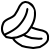Welcome to Lucian's Pedagogy.

This site contains information to help you understand the marking process in humanities, creative arts and science essays.

Please read the Table of Contents (Welcome!). To earn A, (in an assignment, to have a healthy baby or use 50 As to earn a job) please visit the Anarchy Quiz.

Wednesday, December 30, 2009

Closure: Algorithm for the Comment in the Reason

One should either:

Think of a mistake about an algorithm and correct it.

OR

In the previous entry, the astronaut concentrated by sleeping in the sleeping bag. I assumed he slept for 8 hours. Clock proteins decrease as one sleeps. The computer program to calculate the percentage of clock proteins in the brain is as follows.

Suppose one previously thinks of general ideas (e.g. whether the king should give books to the school library). However, a student shouldn't incorrectly follow the rules for board game in a book. Suppose one then thinks of highlights (e.g. to buy sheets of card for Timothy to make a model space station from). However, the launch sequence shouldn't exclude closing the doors causing the toys to fall out and be tripped on. However, suppose one then thinks of specifics (e.g. how to program a space game). However, a person shouldn't throw a tomato, causing someone to retire from a job.

/**

Clock Proteins/4

Clock proteins/4 shows how clock proteins in the brain decrease during sleep.

clock_proteins(+Time1, +Time2, +Graph1, -Graph2)
Clock proteins/4 prints the graph of percentage of clock proteins in the brain over time (h).

(+ means input, - means output)

Clock proteins/4 prints the graph of the percentage of clock proteins in the brain.

Time1 is the current time, for example, 0 hours.
Time2 is the final time, for example, 8 hours.
Graph1 is the current graph, for example, the empty set ([]).
Graph2 is the final graph, for example, [[0, 100], [1, 87.5], [2, 75], [3, 62.5], [4, 50], [5, 37.5], [6, 25], [7, 12.5], [8, 0]], which is the current graph with all the pairs appended to it.

Clock proteins/4 prints the graph of the percentage of clock proteins in the brain from 0 to 8 hours, when the following query is executed.

clock_proteins(0, 8, [], Graph).

At 0 hours, the percentage of clock proteins in the brain is 100% (given by [0, 100]) etc, as given by the following output.

Graph = [[0, 100], [1, 87.5], [2, 75], [3, 62.5], [4, 50], [5, 37.5], [6, 25], [7, 12.5], [8, 0]]

**/

clock_proteins(Time, Time, Graph1, Graph2) :-
%% Clock proteins/4 appends the current time and the percentage which corresponds to it to the current graph to form the final graph, when the current time is equal to the final time.%% For example, clock proteins/4 takes the current time 8 h, the final time 8 h, the current graph [[0, 100], [1, 87.5], ..., [7, 12.5]] and returns the final graph [[0, 100], [1, 87.5], ..., [8, 0]].
append(Graph1, [[Time, 0]], Graph2),
%% Clock proteins/4 appends the current time and the percentage of clock proteins in the brain of 0%, to the current graph to form the final graph.
%% For example, clock proteins/4 appends the current time and percentage of clock proteins in the brain [[8, 0]] to the current graph [[0, 100], [1, 87.5], ..., [7, 12.5]] to form the final graph [[0, 100], [1, 87.5], ..., [7, 12.5], [8, 0]].
!.
%% Clock proteins/4 won't be executed again.
clock_proteins(Time1, Time2, Graph1, Graph2) :-
%% Clock proteins/4 calculates the percentage from 12.5 * (Time2 - Time1) and appends it to the current graph, calculates the other values which form the final graph.
%% For example, clock proteins/4 takes the current time 0 h, the final time 8 h, the current graph [], and returns the final graph [[0, 100], [1, 87.5], ..., [7, 12.5], [8, 0]].
Percentage is 12.5 * (Time2 - Time1),
%% Clock proteins/4 calculates the percentage of clock proteins in the brain, as 12.5 * (the final time - the current time).
%% For example, clock proteins/4 calculates the percentage of clock proteins in the brain 100% from 12.5 * (the final time 8 h - the current time 0 h).
Time3 is Time1 + 1,
%% Clock proteins/4 calculates the new time as the current time + 1 h.
%% For example, clock proteins/4 calculates the new time 1 h as the current time 0 h + 1 h.
append(Graph1, [[Time1, Percentage]], Graph3),
%% Clock proteins/4 appends the time and percentage of clock proteins in the brain to the current graph to form the new graph.
%% For example, clock proteins/4 appends the current time and percentage of clock proteins in the brain [[0, 100]] to the current graph [] to form the new graph [[0, 100]].
clock_proteins(Time3, Time2, Graph3, Graph2).
%% Clock proteins/4 calculates the rest of the times and percentages of clock proteins in the brain from the new time until the final time, takes the new graph and returns the final graph.
%% For example, clock proteins/4 takes the new time 1 h, the final time 8 h, the new graph [[0, 100]] and returns the final graph [[0, 100], [1, 87.5], ..., [7, 12.5], [8, 0]].